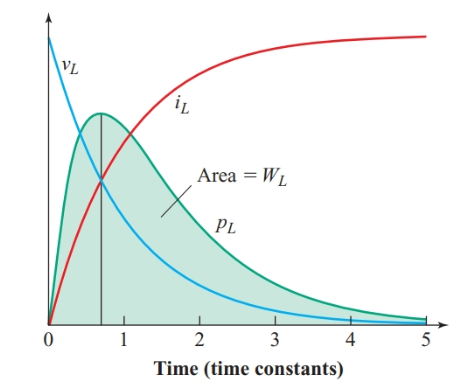# Energy Stored in an Inductor

Want create site? Find Free WordPress Themes and plugins.

If we connect an ideal inductor to a voltage source having no internal resistance, the voltage across the inductance must remain equal to the applied voltage. Therefore, the current rises at a constant rate, as shown in Figure 1(b). The source supplies electrical energy to the ideal inductor at the rate of p = Ei.

Unlike resistance, inductance cannot convert this energy into heat or light. Instead, the energy is stored in the magnetic field as the rising current forces the magnetic lines of force to expand against their tendency to become as short as possible—somewhat as a rubber band stores energy when it is stretched.Figure 1 Determining the energy stored by an inductor

In resistance circuits where the current and voltage do not change with a change in time, the energy transferred from the source to the resistance is W = Pt = VIt.

Although the voltage remains constant in the circuit of Figure 1(a), the current steadily increases as time elapses. However, since the rate of change of current is constant, the average value of the current, I, as it rises from zero to Im is 1/2 Im. Therefore, the energy stored by an inductor as the current rises from zero to Im is

$W=V\times \frac{1}{2}{{\operatorname{I}}_{m}}\times t$

Since the rate of change of current is constant, Inductor voltage becomes

$V=L\frac{di}{dt}=\frac{L{{\operatorname{I}}_{m}}}{t}$

Substituting for V in the equation for W gives

$W=L\times \frac{{{\operatorname{I}}_{m}}}{t}\times \frac{1}{2}{{\operatorname{I}}_{m}}\times t$

$\begin{matrix}W=\frac{1}{2}L\operatorname{I}_{m}^{2} & {} & \left( 1 \right) \\\end{matrix}$

With a practical inductor, both the instantaneous voltage and current are changing and their rates of change are not constant. If we find the voltage across and the current through the inductance for a given moment, we can use relationship p = vi to calculate the rate at which the inductance of the circuit stores energy at that moment. With a series of such calculations, we can plot the graph in Figure 2.Figure 2 Energy stored by a practical inductor

When the current in a practical inductor reaches its steady-state value of Im = E/R, the magnetic field ceases to expand. The voltage across the inductance has dropped to zero, so the power p = vi is also zero. Thus, the energy stored by the inductor increases only while the current is building up to its steady-state value.

When the current remains constant, the energy stored in the magnetic field is also constant. Although no additional energy is stored by the inductance of the practical inductor, the resistance of the inductor dissipates energy at a steady rate of$P=I_{m}^{2}R$. Current must continue to flow to maintain the magnetic field.

The area under the power curve in Figure 2 represents the energy stored by the inductance and is equal to the product of the average power and the elapsed time. The energy stored in the magnetic field of an inductor can be written as:

$\begin{matrix}w=\frac{1}{2}L{{i}^{2}} & {} & \left( 2 \right) \\\end{matrix}$

Where w is the stored energy in joules, L is the inductance in Henrys, and i is the current in amperes.

Example 1

Find the maximum energy stored by an inductor with an inductance of 5.0 H and a resistance of 2.0 V when the inductor is connected to a 24-V source.

Solution

\begin{align}& {{\operatorname{I}}_{m}}=\frac{E}{R}=\frac{24V}{2.0\Omega }=12A \\& W=\frac{1}{2}LI_{m}^{2}=\frac{1}{2}\times 5.0H\times {{\left( 12A \right)}^{2}}=36J \\\end{align}

Did you find apk for android? You can find new Free Android Games and apps.